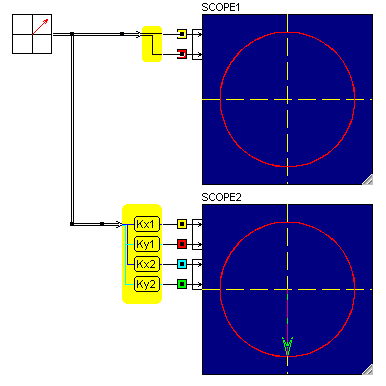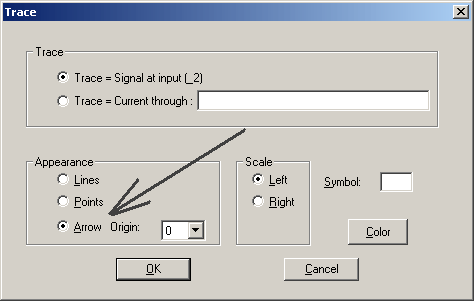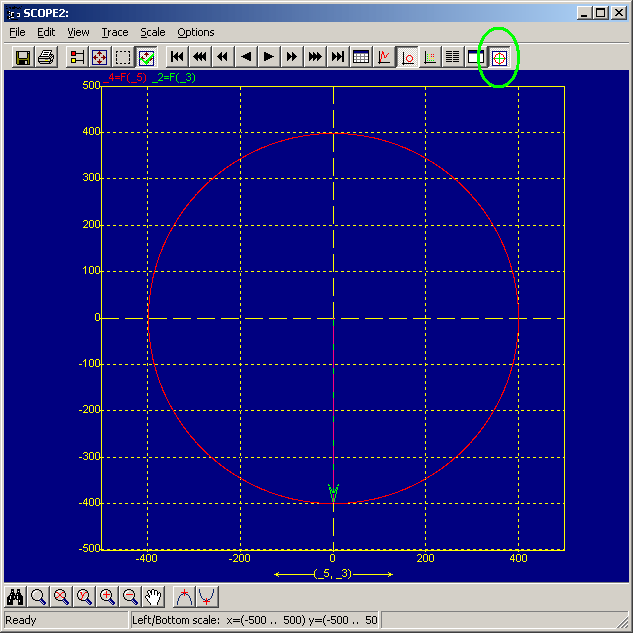• Introduction
• What is in this manual
• What is Caspoc
• User interface
• Introduction
• Starting
• Simulation
• Editing
• Viewing and printing
• Getting Started
• Basic editing
• Simulation in the time domain
• Basic User Interface Topics
• Editing
• Simulation
• Viewing
• Library
• Reports
• Project management
• Circuit and Block Diagram Components
• Introduction
• Cscript and user defined functions
• Component parameters
• Modeling Topics
• Introduction
• Power Electronics
• Semiconductors
• Electrical Machines
• Electrical drives
• Power Systems
• Mechanical Systems
• Thermal Systems
• Magnetic Circuits
• Green Energy
• Coupling to FEM
• Experimenter
• Analog hardware description language
• Embedded C code Export
• Coupling to Spice
• Small Signal Analysis
• Matlab coupling
• Tips and tricks
• Appendices

## Display of vectors in the scope.

The space vector can be displayed in a scope as a phasor. Using the interface component Vector2Scalar the scalar components of the vector are input to the scope. If the scope is in the xy display mode, the space vector will be displayed as a set of lines. If we connect hte spacevector source component tothe scope, we will see a circle.
To additionally display a phasor we can add a second Vector2Scalar component. In the scope select the even trace (in this case number 4) and set the display model to arrow. In the simulation below an interface block ScaledVectorPlot from the Interface/Scope section is used to scale the components of the vector.When displaying in the xy mode, only the properties of even traces have to be set. The trace is also colored with the color specified for the even trace.
To display the space vector as a phasor, select the arrow option for the appearance.In order to have a square scope plot, the size of the scope can be set to a square format. This means that all axis are equal insize aand rotating space vectors are diplayed as a circle.
The scope below shows a circle presentation ofa space vector along with the phasor.